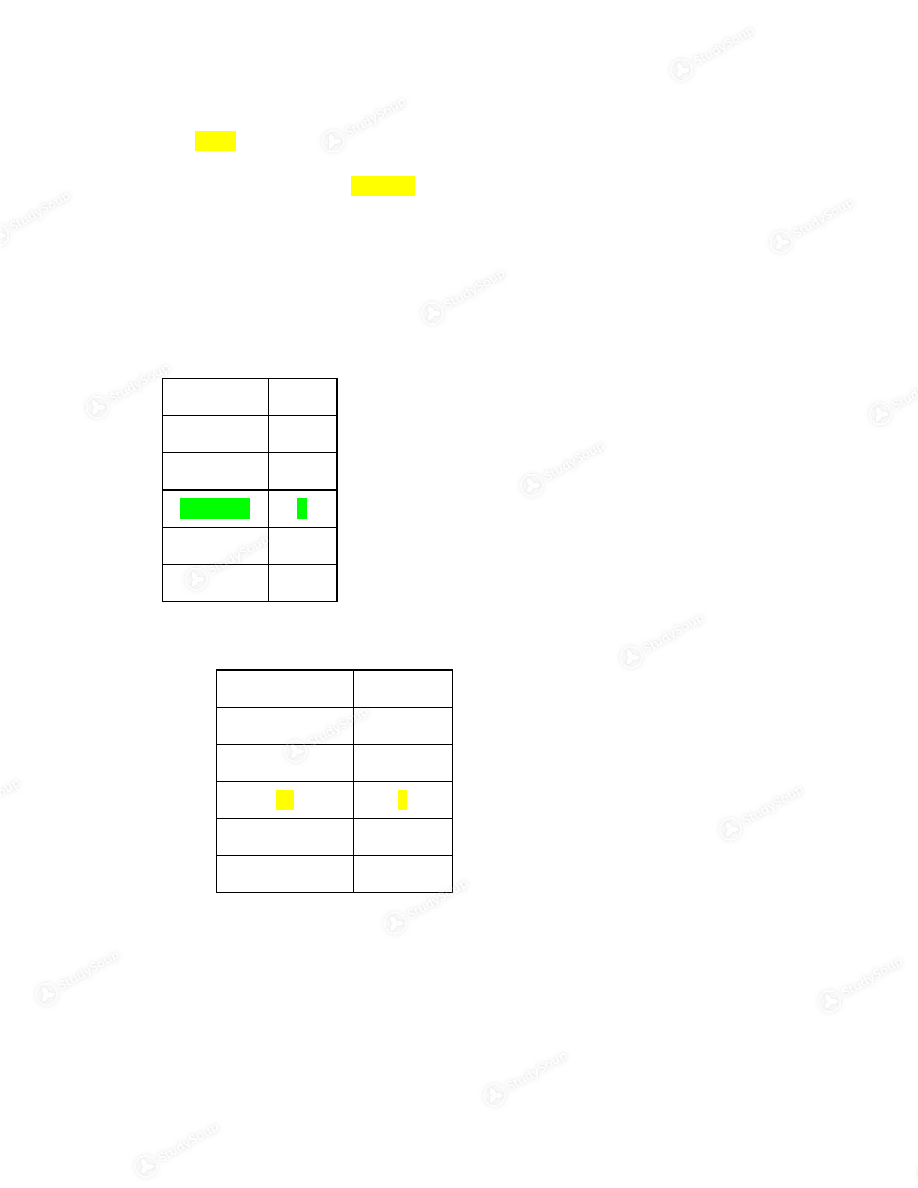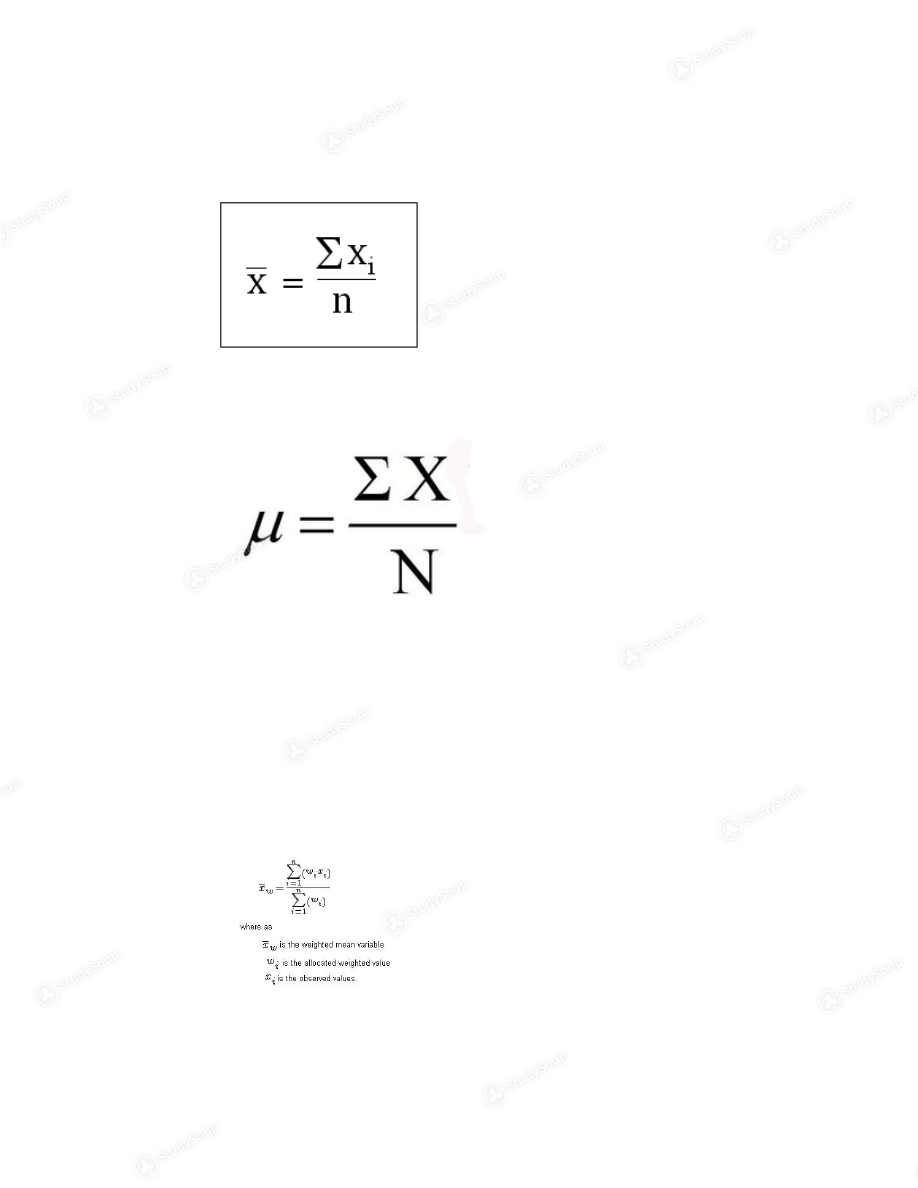×

# IU - SPEA-K 300 - Class Notes - Week 3

### Created by: leesj846 Elite Notetaker

> > > > IU - SPEA-K 300 - Class Notes - Week 3

IU - SPEA-K 300 - Class Notes - Week 3

This preview shows pages 1 - 3 of a 8 page document. to view the rest of the contentThe Mode  ● The  mode  is defined to be the value that occurs most often in a data set
● A data set can have more than one mode (bimodal, unimodal, trimodal)
● A data set is said to have  no mode  if all values occur with equal frequency
● Ex. Data Set: 1,1,2,4,2,5,2
Ordered Set: 1,1,2,2,2,4,5  ○ Mode= 2  ● No Mode  ○ Data Set= 1,2,3,4,5,6  No mode  ● Two modes  ○ Data Set= 1,1,2,2,3,4,5,6  Mode=1,2  ● The mode for a grouped frequency distribution
●
Class  15.5­20.5  20.5­25.5  25.5­30.5  30.5­35.5  35.5­40.5  ○ Modal Class= 25.5­30.5  ● The mode for an ungrouped frequency distribution  ○   Values  15  20  25  30  35  ■ The mode for grouped data is the modal class
■ The modal class is the class with the largest frequency
■ Sometimes the midpoint of the class is used rather than the boundaries
■ Mode=8
● When to use the mode?  ○ When the variable being examined is nominal, only the mode can be used as a  measure of central tendency  ○ When you want to describe the most common value of the distributionThe Median  ● When a data set is ordered, called  data array
● The  median  is defined to be the midpoint of the data array
● The symbol for median=  MD
● There are as many values of the variable below the median as above the median value
○ Ex. 190, 230, 195, 210, 245
○ Data Array: 190, 195, 210, 230, 245
MD= 210  ● In an  odd number  of values in the data set, it is easy to select the middle number in the  data array  ● In an  even number  of values in the data set, the median is obtained by taking the  avg. of  the two middle numbers  ○ Ex. 10, 20, 30, 40, 50, 60
○ Data Array: 10, 20, 30, 40, 50, 60
MD=(30+40)/2= 35  ● The median: Ungrouped Frequency Distribution  ○ For an ungrouped frequency distribution, find the median by examining the  cumulative frequencies to locate the middle value  ○ If ‘n’ is the sample size, compute n/2. Locate the data point where n/2 values fall  below and n/2 values fall above  ○ Ex.   Class  Frequency  ■ To locate the middle point divide 24/2= 12
■ Locate the point where 12 values would fall below and 12 values will fall
above  ■ Consider the cumulative distribution
■ The 12th and 13th value fall in class 2
MD=2  ● When to use the Median?  ○ When the variable of interest is ordinal, internal, or ratio
○ When the mean would a misleading value of the typical case

The Mean
● The mean is defined to be the sum of the data values divided by the total number of  values  ● Think of the mean as the equilibrium of a scale. It balance the data set.● We will compute two means and one for the sample and one for a finite population of  values  ● The mean is not an actual data value
● The sample mean
○ Ex. 1,3,5,7,9  ■ Sample mean= (1+3+5+7+9)/5= 25/5= 5  ● Population Mean   N= Size of the population Mu= Population Mean  ○ Mu= (20,000+40,000+60,000+80,000)/4= 200,000/4= 50,000  ● What does the word “average” mean?  ○ It is ambiguous, since several different methods can be used to obtain an  average  ○ The average means the center of the distribution, or the most typical case in the  data  ○ Measures of average are also called measure of central tendency, and include  the mode, median, mean, and midrange  ● The Weighted Mean  ○ A set of number X1,X2,...,Xn with corresponding weights W1,W2,...,Wn is  computed from the following formula

This is the end of the preview. Please to view the rest of the contentJoin more than 18,000+ college students at Indiana University who use StudySoup to get ahead